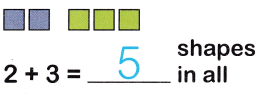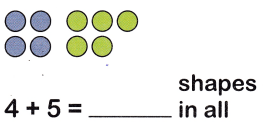# McGraw Hill Math Grade 1 Chapter 2 Lesson 3 Answer Key Addition Facts from 0 to 12

All the solutions provided in McGraw Hill Math Grade 1 Answer Key PDF Chapter 2 Lesson 3 Addition Facts from 0 to 12 as per the latest syllabus guidelines.

## McGraw-Hill Math Grade 1 Answer Key Chapter 2 Lesson 3 Addition Facts from 0 to 12

Use the number line to find how many in all. Write the sum.

Question 1.There are 5 shapes in all.

Question 2.4 + 5 = 9
There are 9 shapes in all.

Question 3.
1 + 0 = ___
By adding 1 and 0 we get 1.

Question 4.
5 + 7 = ___
5 + 7 = 12
By adding 5 and 7 we get 12

Question 5.
5 + 6 = ___
5 + 6 = 11
By adding 5 and 6 we get 11.

Question 6.
6 + 1 = ___
6 + 1 = 7
By adding 6 and 1 we get 7.

Question 7.
4 + 2 = ___